# Technical Brief## ALAMO usage in process optimization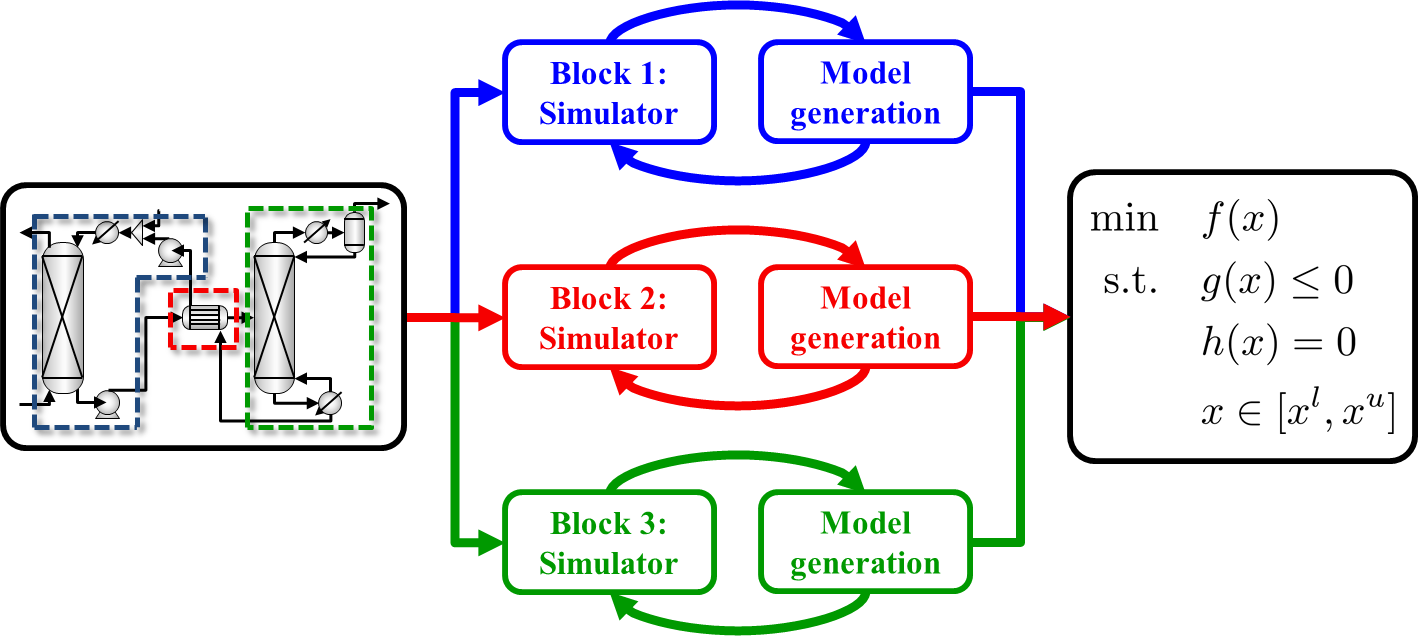In the case of large complex processes, a simulation can be dissaggregated into process blocks. Each block can be modeled separately, then combine with flexible constraints and connectivity variables to generate a final optimization model.

## Notation: Modeling a black-box or process block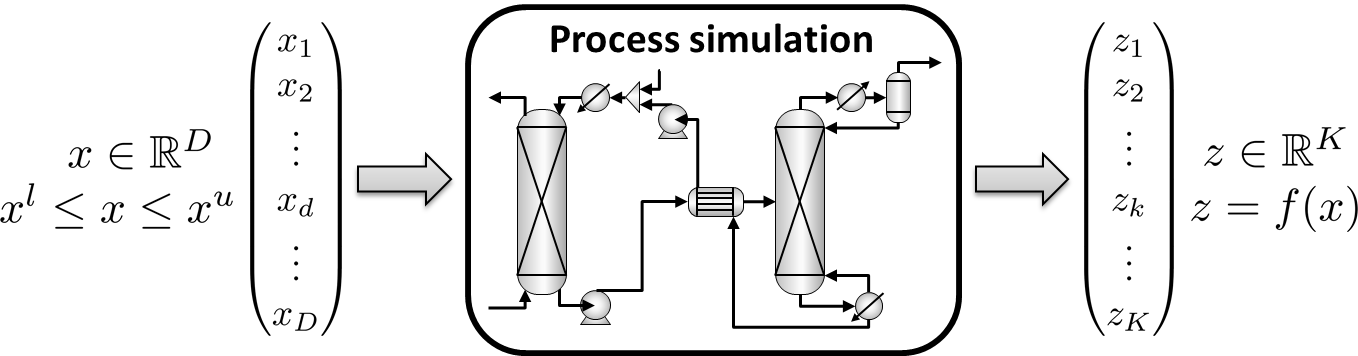Each process block or black-box will have some set of independent variables, x, (operating conditions, inlet flows, etc.) that will be used to define a set of dependent variables, z, (conversions, outlet flows, etc.).

## ALAMO Algorithmic Flowchart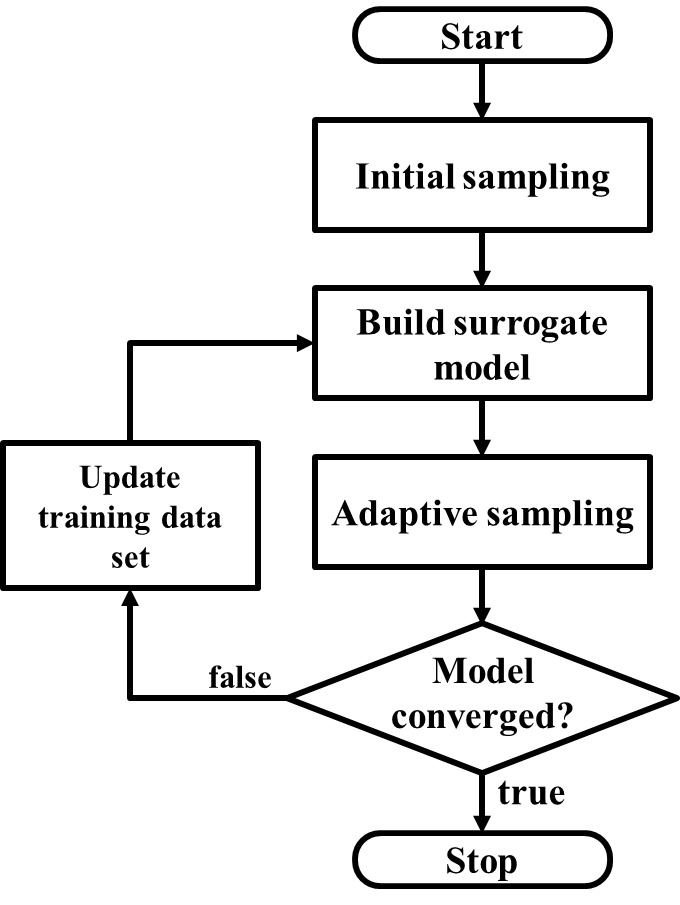For each output variable, z, a surrogate model, zhat(x), will be generated. This will be done in a three step iterative process:

1. Initial design of experiments: Evenly sample the design space to define an initial training set
2. Model building: Build a low complexity surrogate model based on the current training set
3. Adaptive sampling: By interrogating the system using Error Maximization Sampling (EMS), the training set is updated with new points sampled where the current model breaks down.
4. Repeat steps 2 and 3, until no points can be found that violate the model.

In doing this, we aim to generate a model that is

• Accurate
• Simple and compact models for ease the final optimization
• Generated from a minimal data set of simulations or black-box function evaluations

## ALAMO Standard Modeling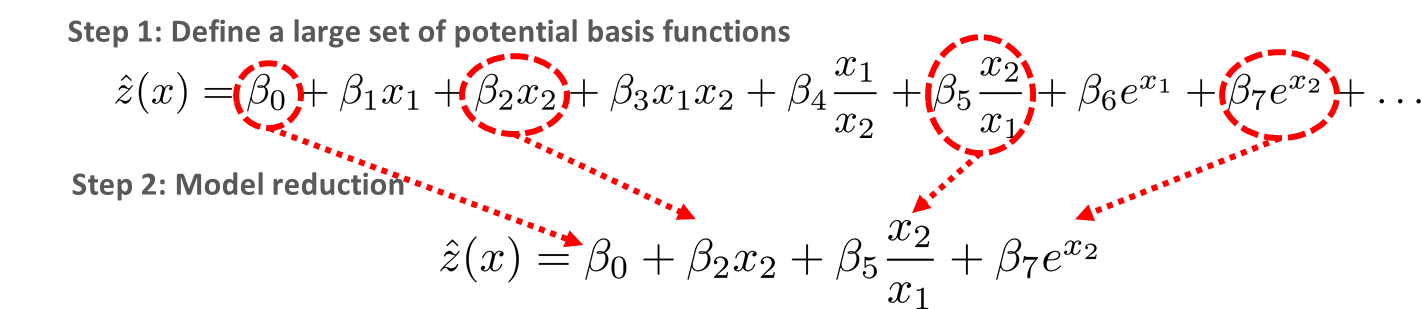ALAMO does not need to know the functional form of the model in advance. To identify the functional form, ALAMO allows for a large set of potential basis functions that can be found from polynomials, engineering experience, physical phenomena, and statistical fitting functions. Next, by combining optimization techniques and statistical methods, we are able to find a subset of basis functions that accurately model the data without overfitting or using unnecessary terms.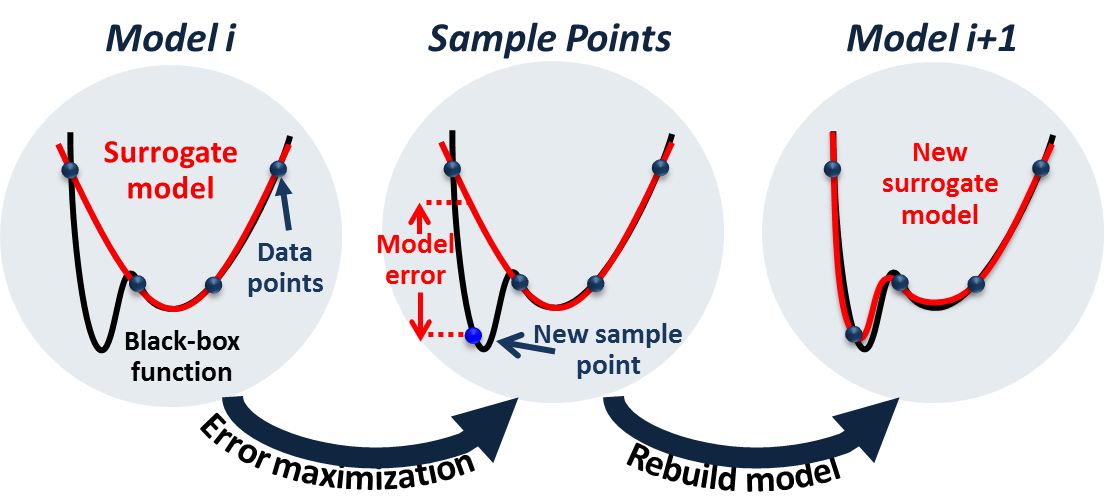After building the current iterate’s model, ALAMO searches the design space to find where our model breaks down using Error Maximization Sampling (EMS). As the name suggests, EMS solves the black-box problem shown below: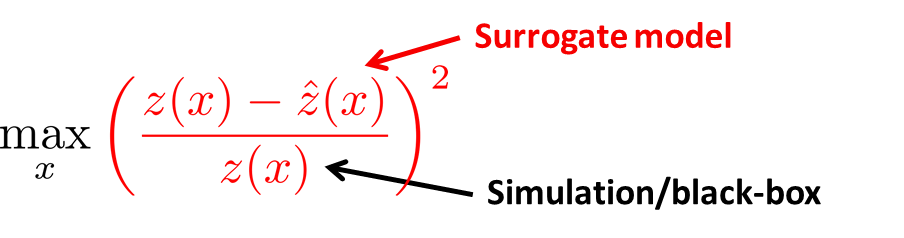Here, the black-box solver SNOBFIT is used to maximize the relative error. During this process, new function evaluations with high model mismatch are added to the training set. ALAMO will then sample the simulation at these new locations, and rebuild the model.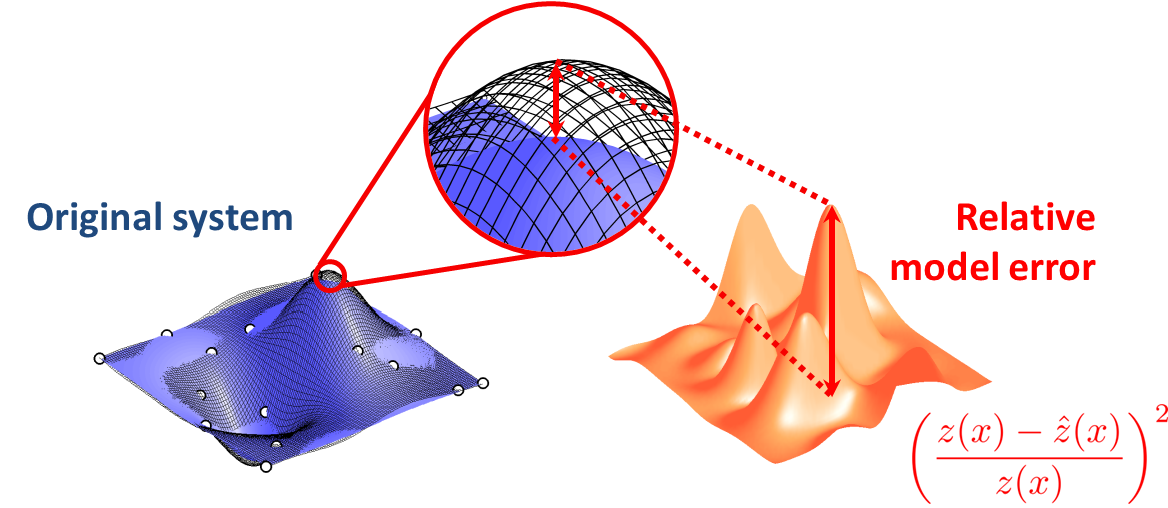If, during this step, no points that violate the model can be found, we will consider our model sufficiently accurate.

## Third party software used

The error maximization adaptive sampling routine in ALAMO uses SNOBFIT, a derivative-free optimization solver. More details about this routine can be found in the SNOBFIT page and the paper: W. Huyer and A. Neumaier, Snobfit -- Stable Noisy Optimization by Branch and Fit, ACM Trans. Math. Software 35 (2008), Article 9. SNOBFIT's quadratic subproblems are solved using MINQ, which is described in: A. Neumaier, MINQ -- General definite and bound constrained indefinite quadratic programming. Web document (1998).